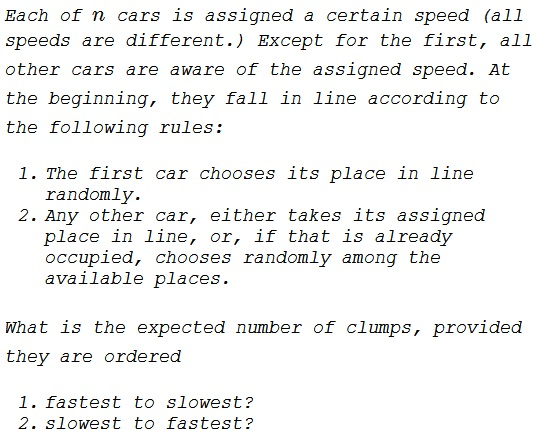# Clumps on a One Lane Road

### Problem 1

Imagine $n$ cars, the drivers of which intend to drive with a specified speeds each. Initially, the cars are queued in uniform random order at the starting point of a semi-infinite, one lane highway. Each car drives at the minimum of its preferred speed and the speed at which the car in front of it is driving. The cars will form "clumps".

What is the expected number of clumps? (Assume all desired speeds are different.)

### Solution 1, Problem 1

Assume that the cars are ranked according to their desired speeds and form a line of the cars randomly, one car at a time, starting with the slowest and proceeding to the fastest. For $k\gt 0,$ let $G(k)$ denotes the number of (potential) clumps accrued so far. Now, the $(k+1)^{st}$ is the fastest among the first $k+1$ cars, so that placing it behind any of the already present $k$ cars would not affect the number of clumps, i.e., in this case, $G(k+1)=G(k),$ and this is with probability $\displaystyle \frac{k}{k+1}.$ The number of clumps will grow by $1:$ $G(k+1)=G(k)+1,$ if the $(k+1)^{st}$ car is placed in front of all the present cars; this happens with probability $\displaystyle \frac{1}{k+1}.$ Thus we arrive at the recurrence

$\displaystyle G(k+1)=\frac{k}{k+1}G(k)+\frac{1}{k+1}\left(G(k)+1\right)=G(k)+\frac{1}{k+1}.$

With $G(1)=1$ and $G(0)=0,$ summing up telescopes to

$\displaystyle G(n)=1+\frac{1}{2}+\frac{1}{3}+\ldots+\frac{1}{n}=H_n,$

the $n^{th}$ harmonic number.

### Solution 2, Problem 1

Again ordering the cars from the slowest to the fastest, a clump leader is a car that's slower than every car ahead of it. Hence $1$ is always a clump leader, $2$ iff it is ahead of $1,$ $3$ iff it is ahead of both $1$ and $2.$ Now expected number of clumps equals expected number of clump leaders which is $\displaystyle G(n)=1+\frac{1}{2}+\frac{1}{3}+\ldots+\frac{1}{n}=H_n.$

### Solution 3, Problem 1

Say we have $N-1$ cars. Add a car to the back. As speeds are random, there is a $\displaystyle \frac{1}{N}$ chance the new car will be the slowest and add one clump. If not, the number of clumps stay the same. So, on average, the $N^{th}$ car adds $\displaystyle \frac{1}{N}$ to the expected number of groups. It's harmonic.

### Solution 4, Problem 1

Recursion. If slowest car is in position $i$ (probability $\displaystyle \frac{1}{n})$ there is one clump behind it plus a field of $i-1$ in front that can again break up into clumps under the same rules. So expectation is

\displaystyle\begin{align}c_n &= 1+\frac{1}{n}\cdot (c_1+\ldots+c_{n-1})\\ & =1+\frac{1}{2}+\frac{1}{3}+\ldots+\frac{1}{n}. \end{align}

### Problem 2### Solution, Problem 2a

... to be continued ...

### Solution, Problem 2b

... to be continued ...

### Acknowledgment

I lifted this problem from Reza Zadeh tweet and several solutions that followed. The reason is two-fold. First off, this is an interesting problem that makes a worthy addition to my collection of probability problems. Second off, most recently I initiated a discussion of the Lost Boarding Pass problem that led to the same kind of answer, the harmonic number $H_n.$ This gave an incentive to seek an extent to which the two problems are related. The wordings are mine.

Solution 1 is by Swapnil Bhatia; Solution 2 is by Christopher D. Long; Solution 3 is by William Heller; Solution 4 is by Markus Meister.